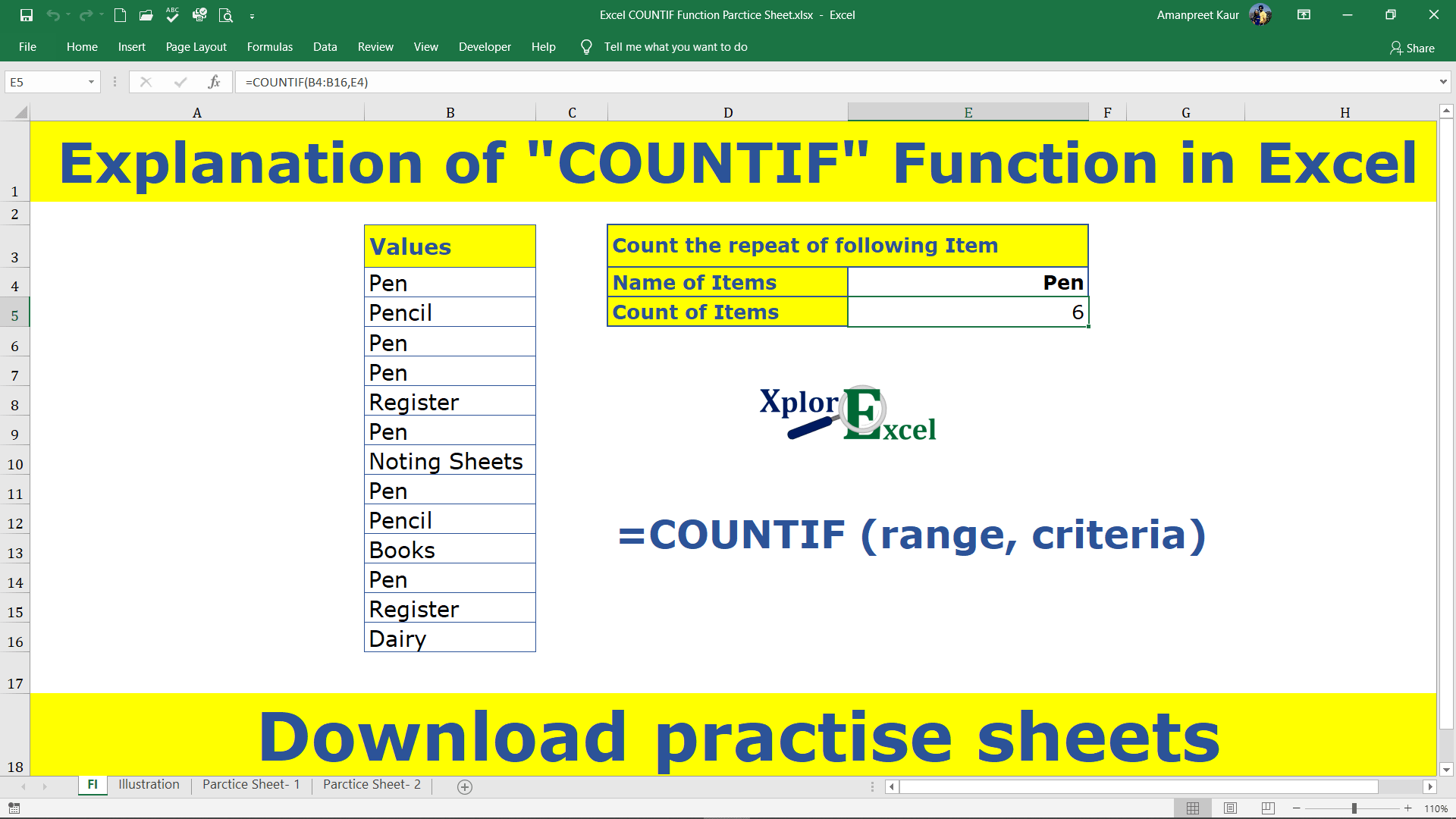## Excel COUNTIF Function: –

The Excel COUNTIF Function is used where we need to count the numbers of repeated values on single criteria. It can count the value of the cells based on a condition of any Text, number, and logical text. It is also working on matching concepts like VLOOKUP and HLOOKUP but with a condition.

### The feature of the “COUNTIF” function: –

• With the help of the COUNTIF Function, we can retrieve the data if the condition is matched with the selected cells.
• It helps in applying a particular condition to the data.

### The logical operator: –

For the “COUNTIF” function we can also use follow logical test on the selected data other than the Text and Number.

 Logical Test Meaning = Equal to <> Not Equal to > Greater than >= Greater than and Equal to < Less than <= Less than and equal to

### Explanation of Function:-

Now, We will explain the Arguments of the Function.

=COUNTIF (range, criteria)

range: – it is that column or row or both of selected cells on which we want to apply the condition or criteria.

criteria: –  This is a condition which we have to apply to the selected cells.

### Example of Function: –

I will show you, how to apply the whole function. (using optional also).

From the following table get the item-wise value of the total sale amount.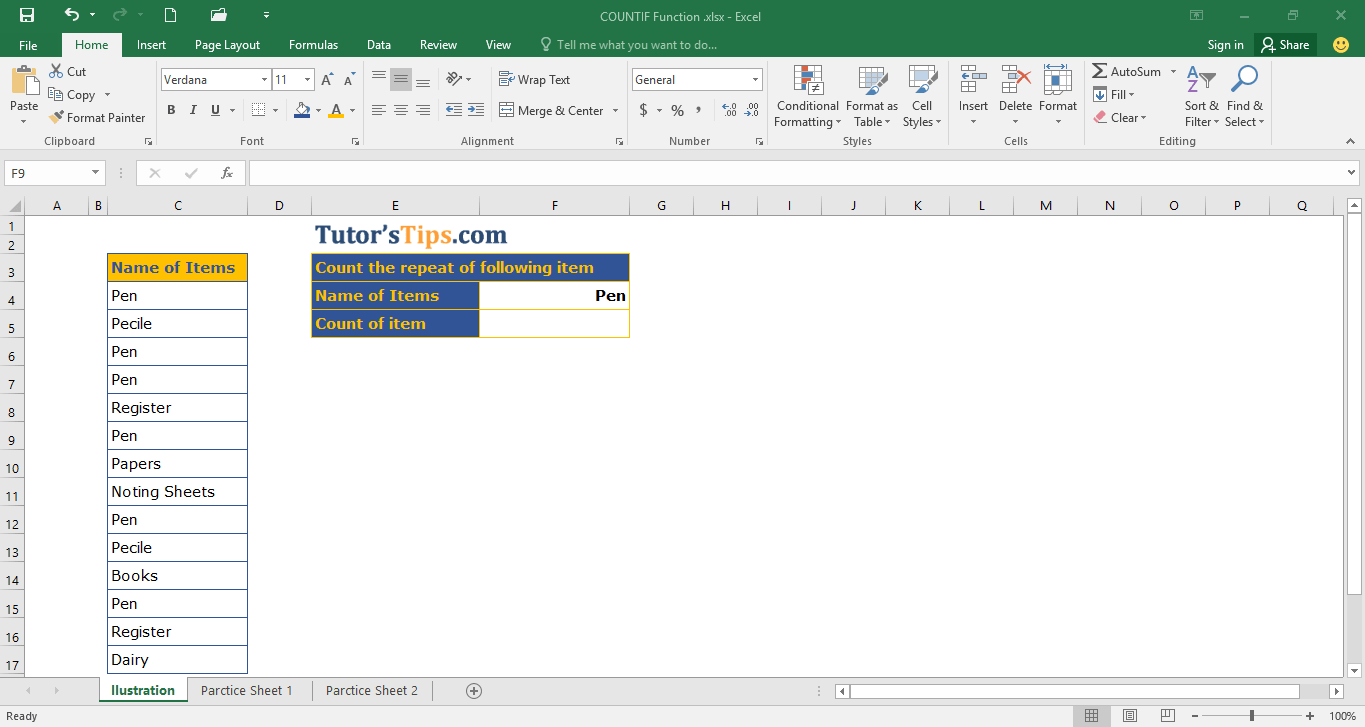Solution:-

We will Apply the COUNTIF function and get the result. This is shown in the following steps: –

Step No. 1:-

We will write the “=COUNTIF( ” in the column of the result.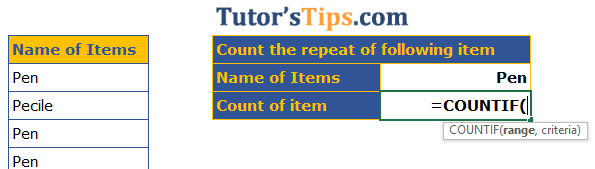Step No. 2

Now select the cells on which you want to apply the Condition or criteria.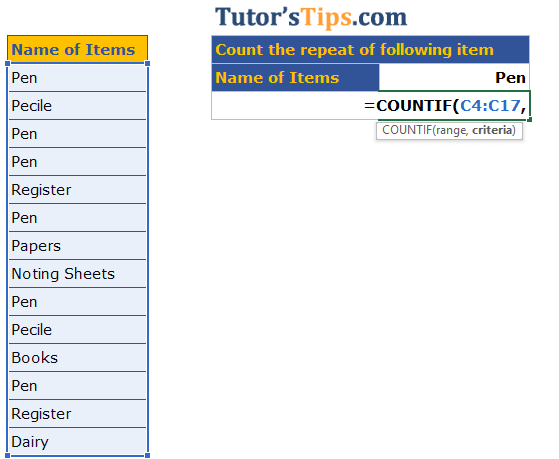Step No. 3

Now select the Condition or criteria which you want to apply.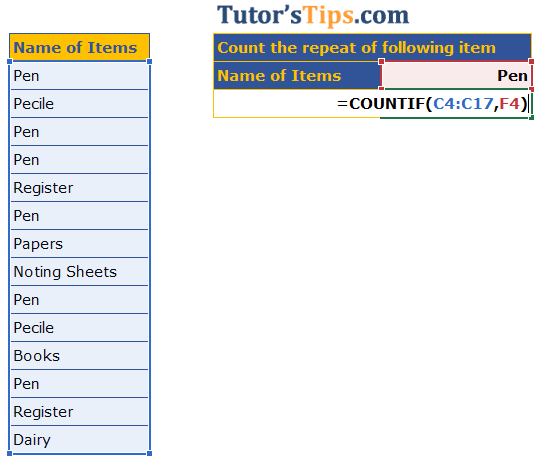Step No. 4: –

Now, Press enter and get the result: –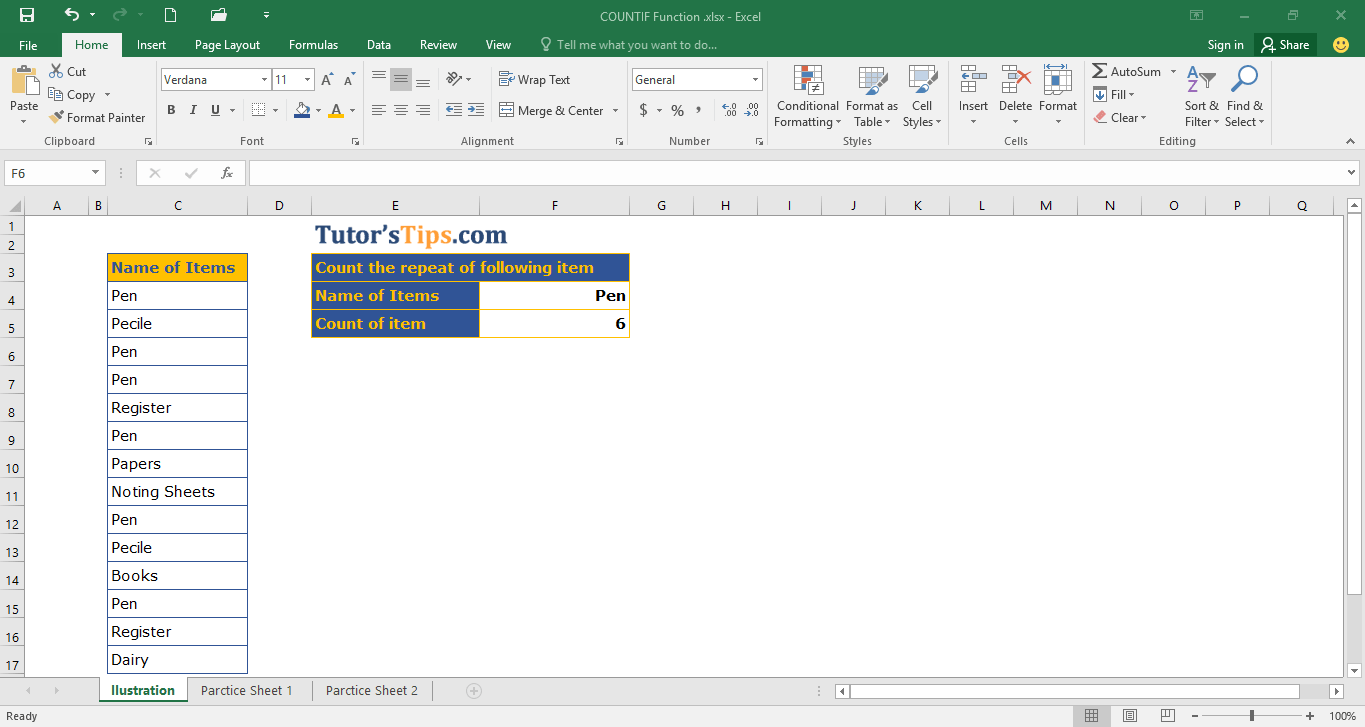Thanks